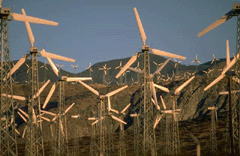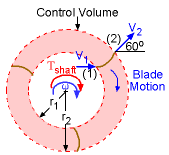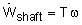Ch 4. Fundamental Laws (Integral Anal.) Multimedia Engineering Fluids ConservationMass LinearMomentum Moment ofMomentum ConservationEnergy
 Chapter 1. Basics 2. Fluid Statics 3. Kinematics 4. Laws (Integral) 5. Laws (Diff.) 6. Modeling/Similitude 7. Inviscid 8. Viscous 9. External Flow 10. Open-Channel Appendix Basic Math Units Basic Equations Water/Air Tables Sections Search eBooks Dynamics Fluids Math Mechanics Statics Thermodynamics Author(s): Chean Chin Ngo Kurt Gramoll ©Kurt GramollFLUID MECHANICS - CASE STUDY IntroductionWindmills A state university is currently working on a long term project to explore the benefits of utilizing wind power as a renewable energy source for the state. Wind comes in at a mass flow rate of 150 kg/s, hits the blades of the windmill horizontally at 20 m/s, and goes out at an angle of 60o at 50 m/s (as shown in the figure). The windmill is rotating at a speed of 2.5 rad/s. Determine the power generated by the windmill. The inner radius, r1, and outer radius, r2, are 30 m and 32 m, respectively. Questions What is the torque and power generated by the windmill?Problem Description (Idealized Flow Conditions) Approach Assume it is a steady-state process. The power generated by the shaft iswhere T is the torque and ω is the angular speed of the shaft.

Practice Homework and Test problems now available in the 'Eng Fluids' mobile app
Includes over 250 problems with complete detailed solutions.
Available now at the Google Play Store and Apple App Store.